# AP Statistics : Graphing Data

## Example Questions

← Previous 1

### Example Question #1 : How To Interpret Dotplots

A basketball coach wants to determine if a player's height can be used to predict the number of points that player scores in a season.  Before using a statistical test to determine the precise relationship of the variables, the coach wants a visual of the data to see if there is likely to be a relationship.  Which of the following should the coach create?

Bar chart

Z-score

Histogram

Bell curve

Scatterplot

Scatterplot

Explanation:

A scatterplot is a diagram that shows the values of two variables and provides a general illustration of the relationship between them.

### Example Question #2 : Graphing Data

Based on the scatter plot below, is there a correlation between theandvariables? If so, describe the correlation.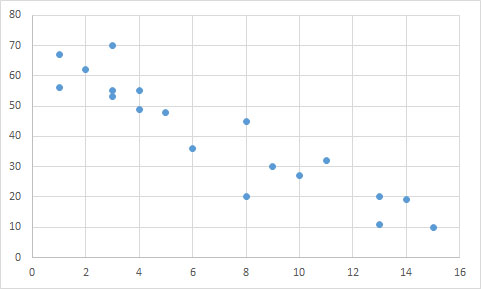Yes; negative exponential relationship

No; there is no correlation

Yes; positive linear relationship

Yes; negative linear relationship

Yes; negative linear relationship

Explanation:

The data points follow an overall linear trend, as opposed to being randomly distributed. Though there are a few outliers, there is a general relationship between the two variables.

A line could accurately predict the trend of the data points, suggesting there is a linear correlation. Since the y-values decrease as the x-values increase, the correlation must be negative. We can see that a line connecting the upper-most and lower-most points would have a negative slope.

An exponential relationship would be curved, rather than straight.

### Example Question #2 : Graphing Data

Order the correlation coefficients to fit the order of the following graphs (two coefficients will not be used),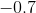,,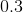,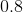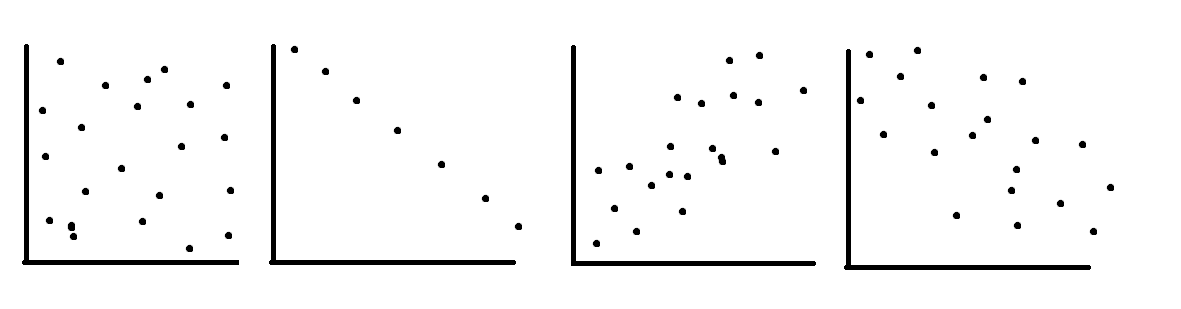,,,,,,,,,,,,Explanation:

The first graph is random scatter, no correlation, the second is perfect linear, corellation, the last two have fairly strong positive and negative corellations, the student should know that a corellation ofis much weaker than them

### Example Question #1 : How To Interpret Stemplots

Find the range of the data in the stem-and-leaf plot.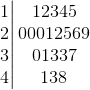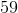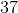Explanation:

To find the range, subtract the minimum value from the maximum value

minimum:maximum:So,

maximum - minimum =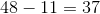### Example Question #2 : How To Interpret Stemplots

Find the median of the stem and leaf plot.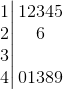Explanation:

The median is the middle value of the set in increasing order.

In this set of 11 entries, the median is the 6th entry of the set in increasing order, or 26.

### Example Question #1 : How To Interpret Stemplots

How many entries are in this stem and leaf plot?Explanation:

The number of entries is the number of digits on the right hand side of the column.

Since there are 21 total digits, there are 21 total entris that make up the stem and leaf plot.

### Example Question #1 : How To Interpret Stemplots

What is the interquartile range of the following data set?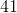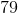Explanation:

This stemplot is read as follows: the stem is the tens digit and each digit in the "leaves" section is a ones digit. Put them together to have a data point.

53, 65, 68, 69, 70, 72, 72, 79, 83, 84, 85, 87, 89, 90, 94

In the particular case there are 15 data points therefore the median is 79. Thus the first quartile is 69 and the third quartile is 87.

Finding the interquartile range is subtracting the first quartile from the 3rd quartile.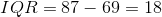### Example Question #1 : How To Interpret Histograms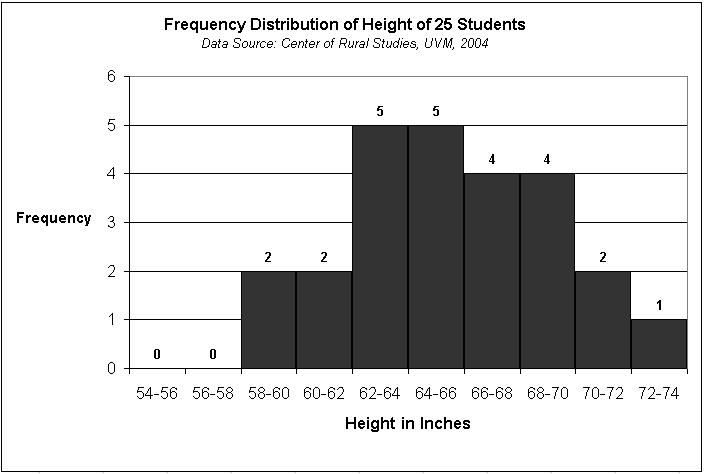Based on the histogram, which set of values was most common in the sample?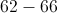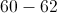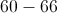Explanation:

Although as a group 62-66 are the most common values in the sample, 60-66 encompasses both 60-62 and 62-66. If you add up the proportion of the sample that is 60-66 inches tall, this proportion is greater than the proportion of the sample that is 60-62 or 62-66 inches tall. Histograms give you the proportion of the sample that has a particular characteristic and you can add up these proportions for a set of characteristics.

### Example Question #1 : How To Interpret Histograms

What is the best measurement of center and spread for the following data set represented by a histogram?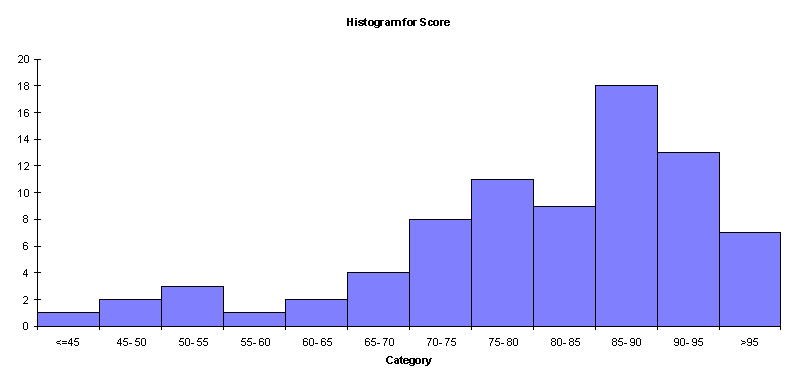Mean and Range

Mean and Standard Deviation

Mean and Interquartile Range

Median and Interquartile Range

Median and Standard Deviation

Median and Interquartile Range

Explanation:

For skewed distributions, the outliers can greatly affect the value of the mean and the standard deviation. Therefore, the median and IQR would be better measures of center and spread because they are not greatly influenced by outlier values.

### Example Question #1 : Graphing Data

Louie collects data on the amount of sleep students in his class get.

Below is a graphical representation of the data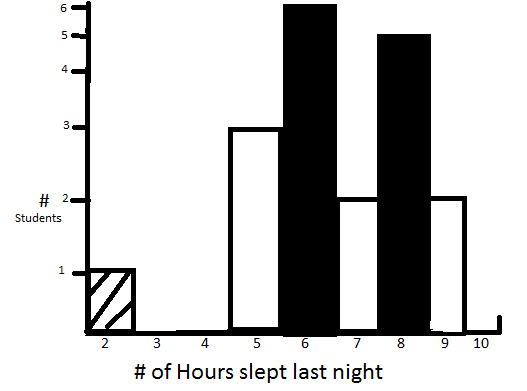Which of the following is/are false about Louie's class' data?

i: the student with 2 hours of sleep is an outlier

ii: without the student with 2 hours of sleep the data is roughly normally distributed

iii: without the student with 2 hours of sleep the data is slightly right skewed

iv: the student with 2 hours of sleep is within 2 standard deviations of the mean

ii & iv

ii, iii & iv

i & ii

i, ii & iii

iii & iv

ii & iv

Explanation:

Entering the data into a calculator you can quickly find the mean to be 6.5 and the sd to be 1.6 6.5-3.2=3.3 3.3>2 so iv is false and by virtue of this 2 is an outlier so i is true.

Between ii and iii, the data is not bell shaped, and does exhibit a slight right skew without the mentioned pt due to more density on 5 and 6 hours than 8 and 9

← Previous 1

### All AP Statistics Resources